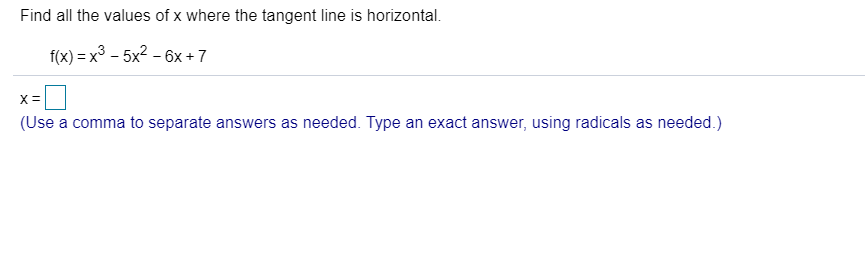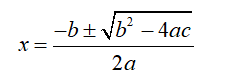# Find all the values of x where the tangent line is horizontal.f(x) x3 -5x2-6x +7X =(Use a comma to separate answers as needed. Type an exact answer, using radicals as needed.)

Question
6 viewshelp_outlineImage TranscriptioncloseFind all the values of x where the tangent line is horizontal. f(x) x3 -5x2-6x +7 X = (Use a comma to separate answers as needed. Type an exact answer, using radicals as needed.) fullscreen
check_circle

Step 1

Horizontal tangent line is located where the derivative of the function takes zero value.

Here the function is f (x) = x3-5x2-6x+7

For finding the value of x use the derivate of the function to locate the zero.

Step 2

Now the derivative of the function:

Step 3

Now for finding the value of x put f’(x) =0 .

3x2-10x-6=0

It’s a quadratic equation of the form ax2+bx+c =0 so the solutions are...

### Want to see the full answer?

See Solution

#### Want to see this answer and more?

Solutions are written by subject experts who are available 24/7. Questions are typically answered within 1 hour.*

See Solution
*Response times may vary by subject and question.
Tagged in

### Calculus